# RD Sharma Solutions for Class 11 Chapter 3 - Functions Exercise 3.2

Exercise 3.2 of Chapter 3, deals with real functions and problems based on it. Students are advised to practice the problems from the RD Sharma textbook keeping aside RD Sharma Class 11 Solutions developed by experts for revision purpose. Students who aim to secure good marks in their exams should practice the problems regularly without fail. Here, the solutions to this exercise are provided in pdf format, which can be downloaded easily from the links given below and students can start practising offline.

## Download the Pdf of RD Sharma Solutions for Class 11 Maths Exercise 3.2 Chapter 3 – Functions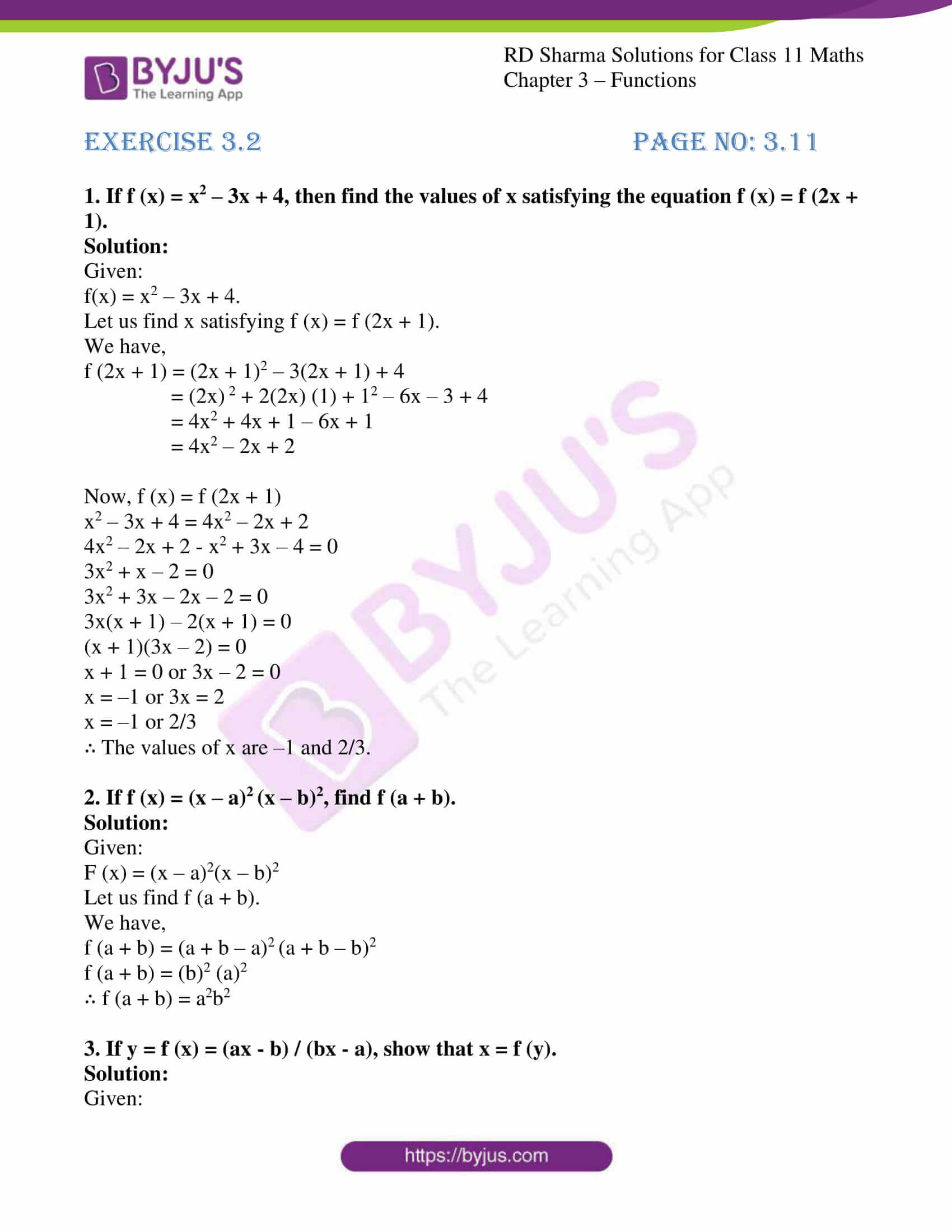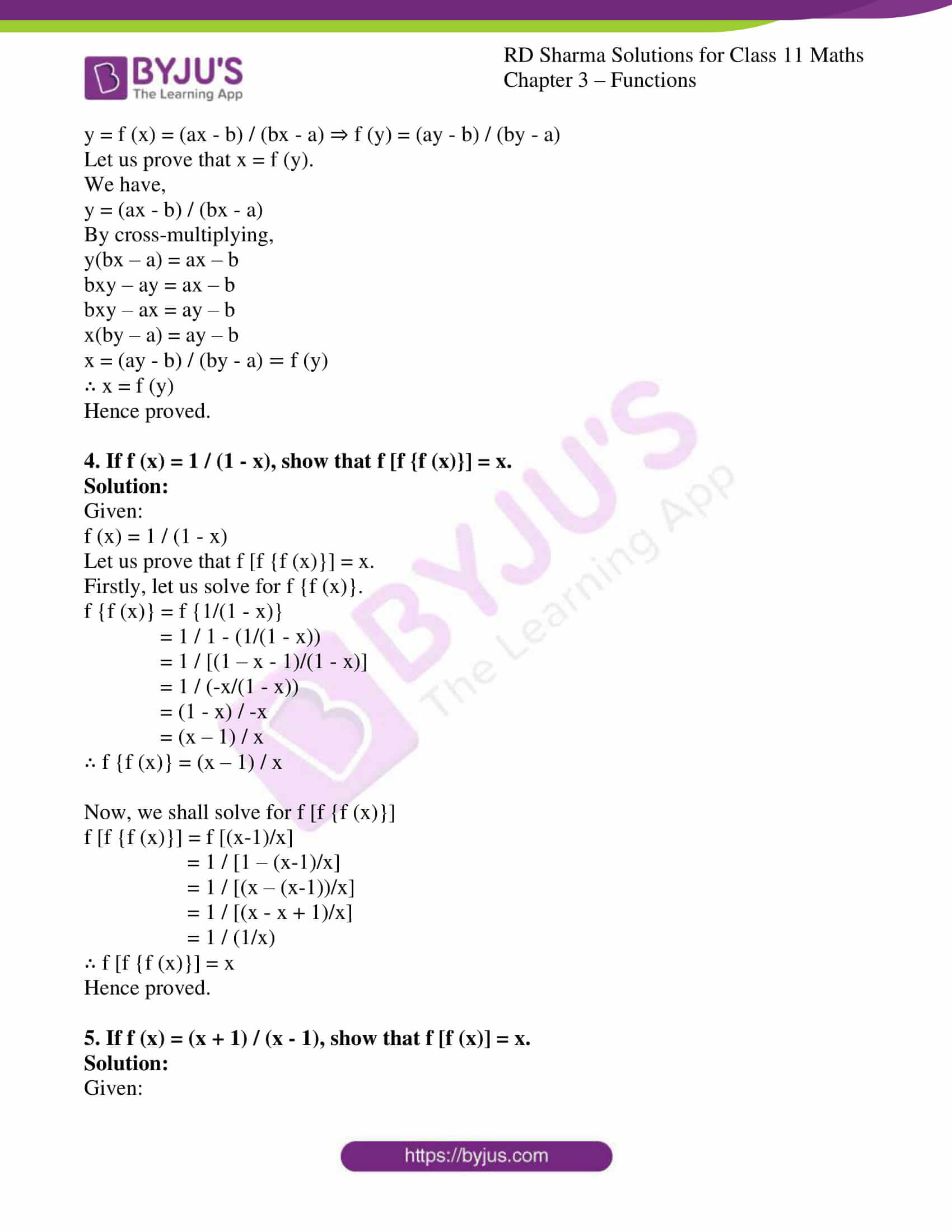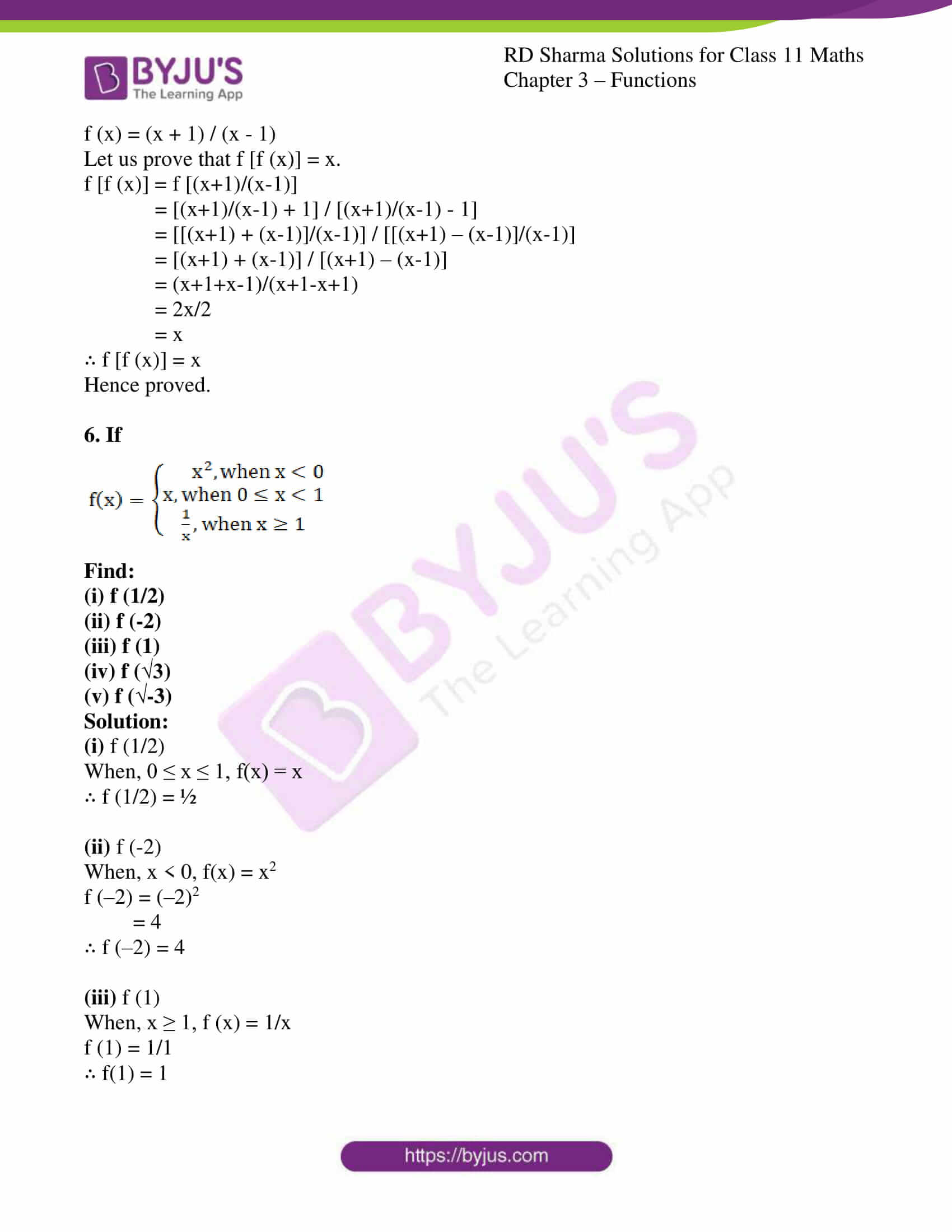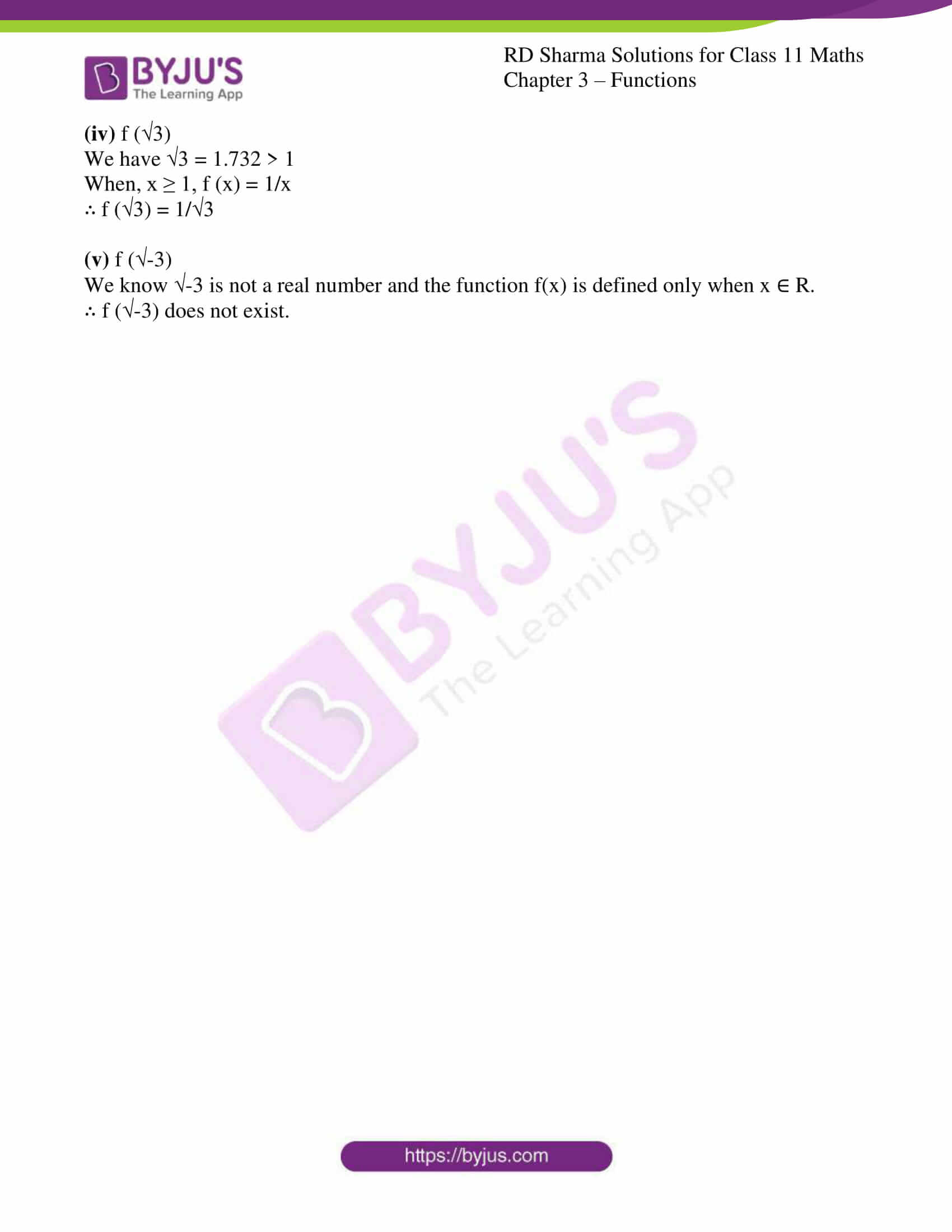### Access answers to RD Sharma Solutions for Class 11 Maths Exercise 3.2 Chapter 3 – Functions

1. If f (x) = x2 – 3x + 4, then find the values of x satisfying the equation f (x) = f (2x + 1).

Solution:

Given:

f(x) = x2 – 3x + 4.

Let us find x satisfying f (x) = f (2x + 1).

We have,

f (2x + 1) = (2x + 1)2 – 3(2x + 1) + 4

= (2x) 2 + 2(2x) (1) + 12 – 6x – 3 + 4

= 4x2 + 4x + 1 – 6x + 1

= 4x2 – 2x + 2

Now, f (x) = f (2x + 1)

x2 – 3x + 4 = 4x2 – 2x + 2

4x2 – 2x + 2 – x2 + 3x – 4 = 0

3x2 + x – 2 = 0

3x2 + 3x – 2x – 2 = 0

3x(x + 1) – 2(x + 1) = 0

(x + 1)(3x – 2) = 0

x + 1 = 0 or 3x – 2 = 0

x = –1 or 3x = 2

x = –1 or 2/3

∴ The values of x are –1 and 2/3.

2. If f (x) = (x – a)2 (x – b)2, find f (a + b).

Solution:

Given:

F (x) = (x – a)2(x – b)2

Let us find f (a + b).

We have,

f (a + b) = (a + b – a)2 (a + b – b)2

f (a + b) = (b)2 (a)2

∴ f (a + b) = a2b2

3. If y = f (x) = (ax – b) / (bx – a), show that x = f (y).

Solution:

Given:

y = f (x) = (ax – b) / (bx – a) ⇒ f (y) = (ay – b) / (by – a)

Let us prove that x = f (y).

We have,

y = (ax – b) / (bx – a)

By cross-multiplying,

y(bx – a) = ax – b

bxy – ay = ax – b

bxy – ax = ay – b

x(by – a) = ay – b

x = (ay – b) / (by – a) = f (y)

∴ x = f (y)

Hence proved.

4. If f (x) = 1 / (1 – x), show that f [f {f (x)}] = x.

Solution:

Given:

f (x) = 1 / (1 – x)

Let us prove that f [f {f (x)}] = x.

Firstly, let us solve for f {f (x)}.

f {f (x)} = f {1/(1 – x)}

= 1 / 1 – (1/(1 – x))

= 1 / [(1 – x – 1)/(1 – x)]

= 1 / (-x/(1 – x))

= (1 – x) / -x

= (x – 1) / x

∴ f {f (x)} = (x – 1) / x

Now, we shall solve for f [f {f (x)}]

f [f {f (x)}] = f [(x-1)/x]
= 1 / [1 – (x-1)/x]

= 1 / [(x – (x-1))/x]

= 1 / [(x – x + 1)/x]

= 1 / (1/x)

∴ f [f {f (x)}] = x

Hence proved.

5. If f (x) = (x + 1) / (x – 1), show that f [f (x)] = x.

Solution:

Given:

f (x) = (x + 1) / (x – 1)

Let us prove that f [f (x)] = x.

f [f (x)] = f [(x+1)/(x-1)]

= [(x+1)/(x-1) + 1] / [(x+1)/(x-1) – 1]

= [[(x+1) + (x-1)]/(x-1)] / [[(x+1) – (x-1)]/(x-1)]

= [(x+1) + (x-1)] / [(x+1) – (x-1)]

= (x+1+x-1)/(x+1-x+1)

= 2x/2

= x

∴ f [f (x)] = x

Hence proved.

6. If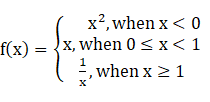Find:

(i) f (1/2)

(ii) f (-2)

(iii) f (1)

(iv) f (√3)

(v) f (√-3)

Solution:

(i) f (1/2)

When, 0 ≤ x ≤ 1, f(x) = x

∴ f (1/2) = ½

(ii) f (-2)

When, x < 0, f(x) = x2

f (–2) = (–2)2

= 4

∴ f (–2) = 4

(iii) f (1)

When, x ≥ 1, f (x) = 1/x

f (1) = 1/1

∴ f(1) = 1

(iv) f (√3)

We have √3 = 1.732 > 1

When, x ≥ 1, f (x) = 1/x

∴ f (√3) = 1/√3

(v) f (√-3)

We know √-3 is not a real number and the function f(x) is defined only when x ∈ R.

∴ f (√-3) does not exist.